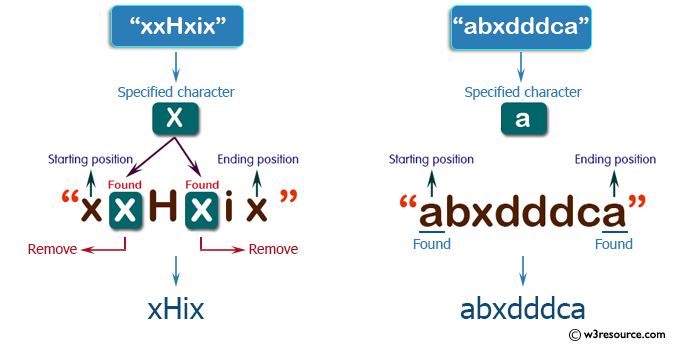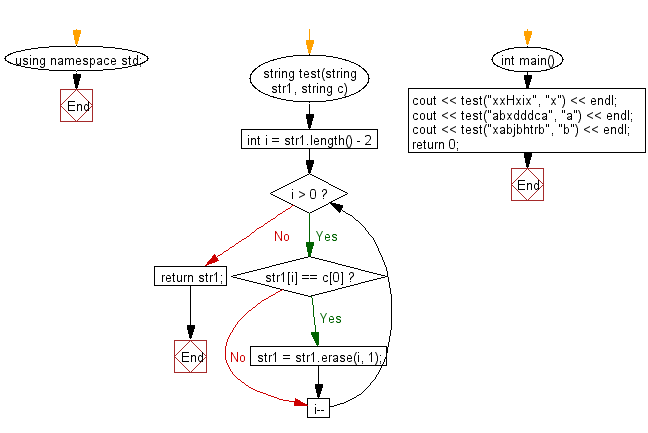﻿ C++ : Delete an element of a string except for the start,end# C++ Exercises: Create a new string from a given string where a specified character have been removed except starting and ending position of the given string

## C++ Basic Algorithm: Exercise-33 with Solution

Write a C++ program to create a new string from a given string where a specified character have been removed except starting and ending position of the given string.

Sample Solution:

C++ Code :

``````#include <iostream>

using namespace std;

string test(string str1, string c)
{
for (int i = str1.length() - 2; i > 0; i--)
{
if (str1[i] == c)
{
str1 = str1.erase(i, 1);
}
}

return str1;
}

int main()
{
cout << test("xxHxix", "x") << endl;
cout << test("abxdddca", "a") << endl;
cout << test("xabjbhtrb", "b") << endl;
return 0;
}
``````

Sample Output:

```xHix
abxdddca
xajhtrb
```

Pictorial Presentation:Flowchart:C++ Code Editor:

Contribute your code and comments through Disqus.

What is the difficulty level of this exercise?

﻿